Handout Exercises (to be presented in class on Thursday, September 22)

Exercise 1.  The linear congruence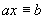(mod m) has a solutionif and only if the equation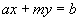has a solution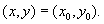Exercise 2.  The linear congruence(mod m) has at least one solution if and only if b is a multiple of gcd(a, m).

Exercise 3.  If gcd(a, m) = 1, then prove that the linear congruence(mod m) has a unique solution.

Exercise 4.  If gcd(a, m) = d and d | b, then prove that the linear congruence(mod m) has exactly d solutions.  (Hint: If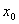is a solution to the linear congruence, then  show that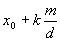,  k = 0, 1, 2, 3, …, d-1, is a solution too. What if k > d?)

Exercise 5.

a) Let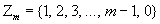be the set of all possible remainders modulo m.  Which elements in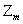have multiplicative inverses?  That is, for which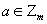does there exist a solution to the equation: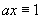(mod m)?  Explain. (NOTE: In the situation where a solution of(mod m) exists, it is typically denoted by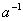.)

b) Let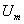denote the set of elements inthat have multiplicative inverses.  Prove that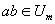whenever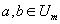.

Exercise 6.  Prove that if gcd(c, m) = 1, then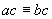(mod m) implies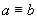(mod m).

Back to Block 1 Course Assignments